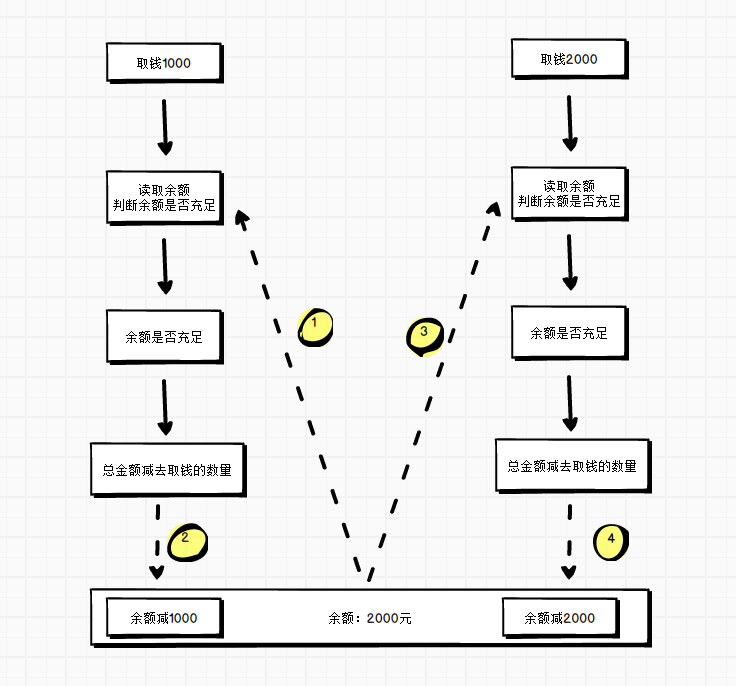并发问题

并发问题public class AppTest {
private int count = 0;
public static void main(String[] args) {
long ts = System.currentTimeMillis();
AppTest app = new AppTest();

for (int i = 0; i < 10000; i++) {
for (int m = 0; m < 1000; m++) {
app.count();
}
});
}
//启动线程
for (Thread t : tList) {
t.start();
}
//等待所有线程执行完
for (Thread t : tList) {
try {
t.join();
} catch (InterruptedException e) {
e.printStackTrace();
}
}

System.out.println(app.count);
long tend = System.currentTimeMillis();
System.out.println(tend-ts);
}

private  void count() {
count++;
}
}



解决并发

使用锁 synchronized


private synchronized void count() {
count++;
}



使用CAS操作

private AtomicInteger count = new AtomicInteger();

private void count() {
}



(本文完)